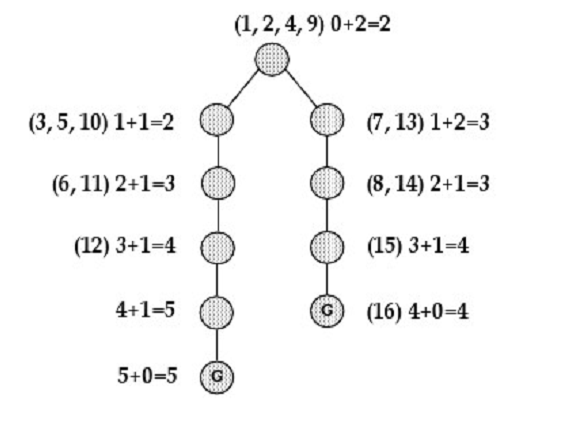# Iterative Deepening A* Star Explanation

1 view

edited

Can somebody explain about Iterative Deepening A*? I still don't understand how it works. Iterative deepening search w/ Depth First Search, and If still not found the solution; increase the Depth++ until found solution.

If Iterative deepening using Depth, then Iterative Deepening A* use what to limit their search?

Here is a picture if you need to explain how it IDA* Works, I just don't understand how it works.

(1,2,4,9) and etc is the step

0+2=2 is f(n)=g(n)+h(n)by (57.5k points)

Iterative deepening A* (IDA*) is a graph traversal and path search algorithm that can find the shortest path between a designated start node and any member of a set of goal nodes in a weighted graph. It is an alternative of iterative deepening depth-first search that borrows the idea to use a heuristic function to evaluate the remaining cost to get to the goal from the A* search algorithm. As you can get, it is a depth-first search algorithm, its memory usage is weaker than in A*, but unlike ordinary iterative deepening search, it concentrates on exploring the most likely nodes and thus does not go to the same depth everywhere in the search tree. Unlike A*, IDA* does not use dynamic programming and therefore often ends up exploring the same nodes many times.